# Add or edit column formulas

The Add Column and Edit Column Pipeline steps allow you to use formulas to transform your data. You can either use one of our built-in formulas as-is, or write your own formula using the Custom formula option.

Note: If you aren’t seeing a formula type you expect to see for an existing column, check the column’s data type. Some formulas are only available for numeric columns.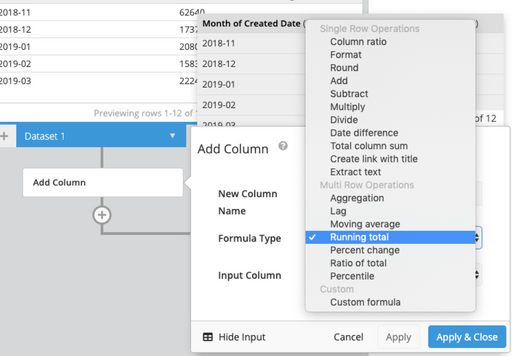Tip: When dividing integers, be sure to multiply the top value by 1.0 to convert your results to a decimal. For example:

``````(1.0 * "Count of Clicks")/"Ad Cost"
``````

Formulas
Single Row Operations Multi Row Operations Custom
Column Ratio Aggregation Custom Formula
Format Lag
Round Moving Average
Substract Percent Change
Multiply Ratio of Total
Divide Percentile
Date Difference
Total Column Sum
Extract Text

## Single Row Operations

### Column Ratio

Easily divide one column by another to get a ratio. Integers are auto-converted to decimals to ensure decimal accuracy.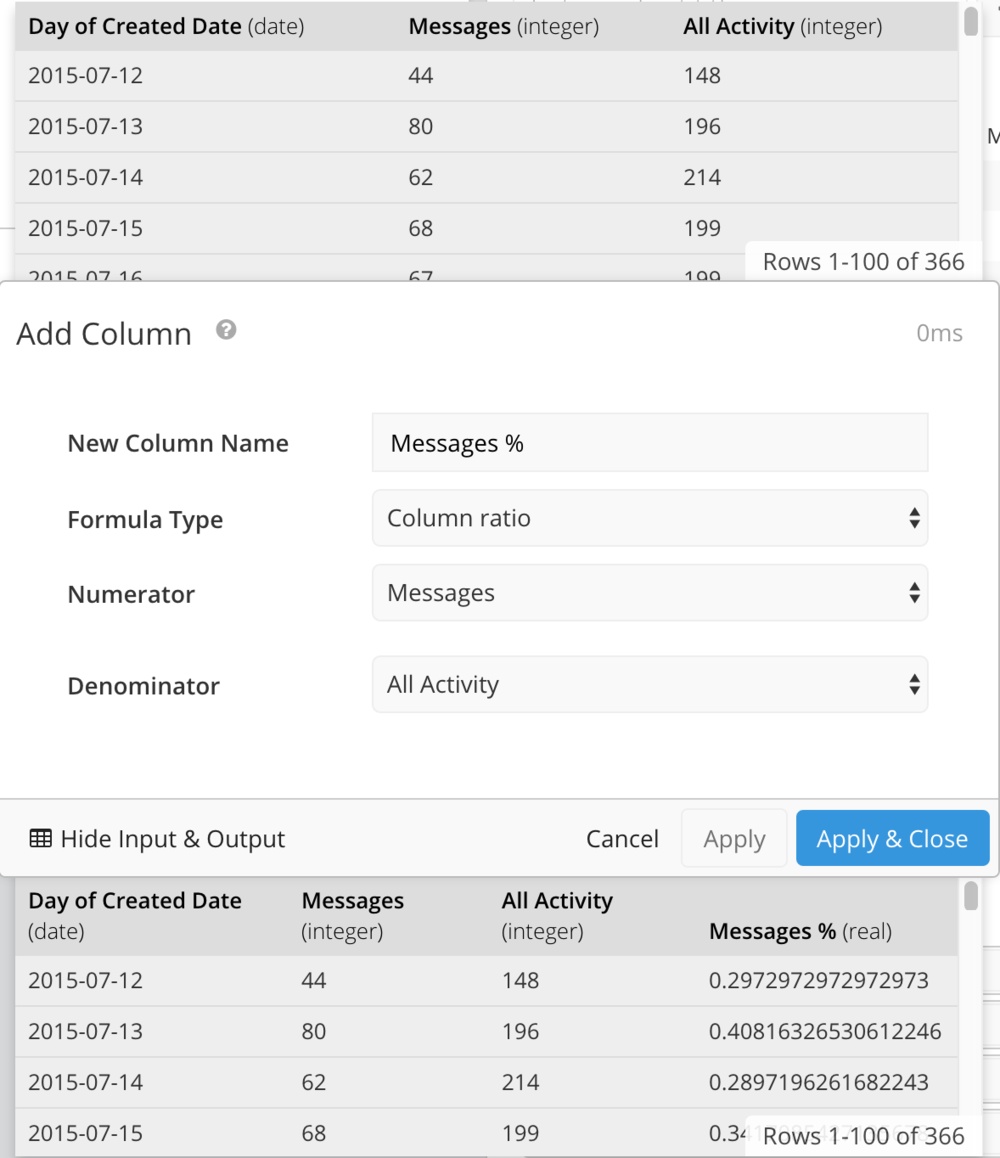### Format

Used to specify decimal precision, useful for force-formatting currency. Format returns a string, not an integer. Therefore, it is best used in table charts or Single Value charts.

### Round

Round is used to round to a specific number of decimal places.

Note: You cannot round a number to a higher decimal precision than it already has. For example, you cannot round the integer 10 to have two decimal places.

Add a numerical value to a column. Enter a column name to add a column value instead.

### Subtract

Subtract a value from a column. You may also subtract a column from another column.

### Multiply

Multiply a column by a value, or by another column.

### Divide

Divide a column by a value, or by another column.

### Date Difference

Calculate the amount of time between two dates. Choose which time unit the value is calculated in: days, weeks, months, etc.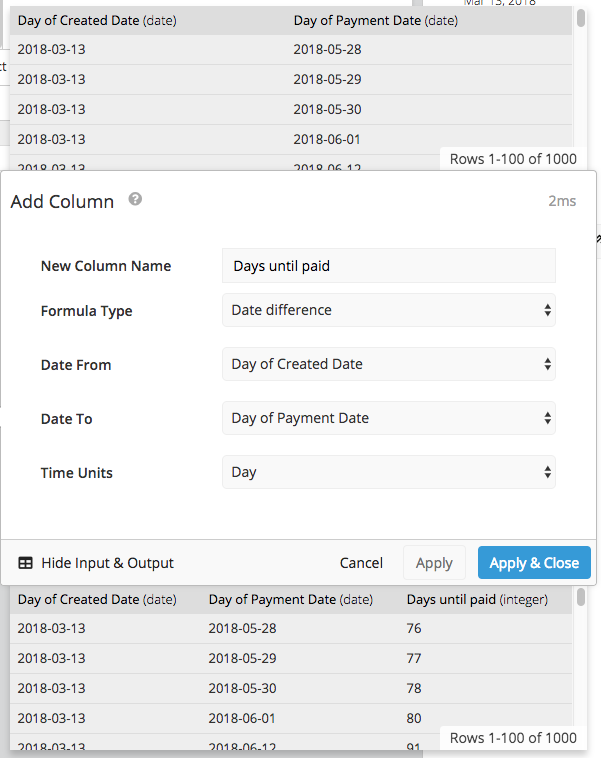### Total Column Sum

Creates a new column where each value is a sum of its row.

Takes a URL column and a text column and generates a markdown link that’s clickable from your table chart, using the text column as the link title.

Useful for creating short, easily parsable links.### Extract Text

Select a specific part of a string and either make the substring a new column (Add Column step) or replace the existing column data (Edit Column step). Choose the starting index (1 starts with the first letter), and then choose either a length or check “To the end” to include all characters to the end of the string.## Multi Row Operations

### Aggregation

Creates a new column with an aggregation value of another column. Choose from AVG, COUNT, MAX, MEDIAN, MIN, or SUM.

Useful for applying calculations to a column that involve an aggregated value. For example, use SUM aggregation, then Column Ratio in the Add Column pipeline step to determine a column value’s percentage of the total column.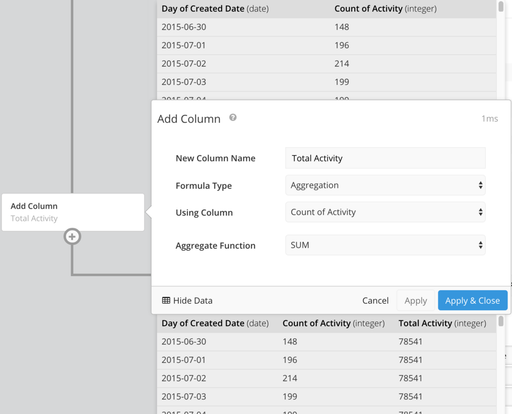### Lag

Access data from a previous row in the same column. Row offset defines how many rows back you want to use.

### Moving Average

Generates a series of averages of your data to create a smooth trend line. Trailing rows is the number of previous rows to include in each row’s average.

### Running Total

Cumulative sum of all previous results of a column.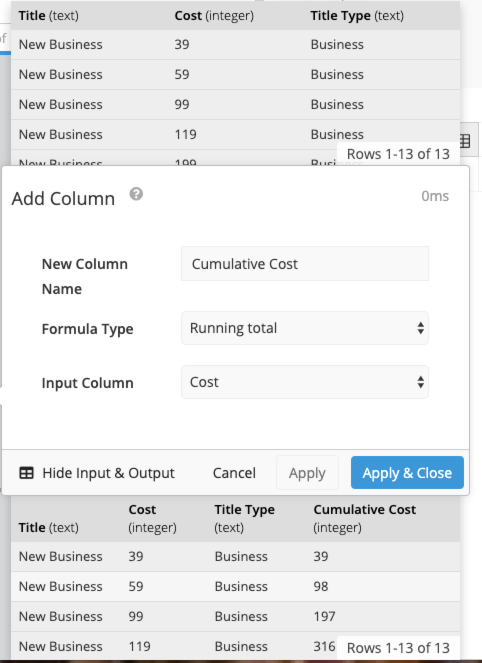### Percent Change

Determines percent change between current and previous row.

### Ratio of Total

Displays each row’s value as a ratio of the column sum.

### Percentile

Determines the percentile of each value in a numeric column. For example, a value is at the 50th percentile if half of the values in the column are less than or equal to it.

## Custom

### Custom Formula

Perform basic calculations on your column, or combine one or more of our formulas as needed. SQLite functions are also supported. See the SQLite documentation for the full list of core functions, aggregate functions, and date and time functions available.

#### Math Functions

acos, asin, atan, atn2, atan2, acosh, asinh, atanh, difference, degrees, radians, cos, sin, tan, cot, cosh, sinh, tanh, coth, exp, log, log10, power, sign, sqrt, square, ceil, floor, pi